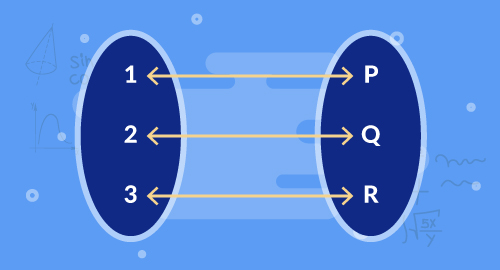#### Need Help?

Get in touch with us1-646-564-2231SAT/ACT

# Functions

Maths

Understand that a function has exactly one output for each input. The graph of the function is the set of ordered pairs consisting of an input and corresponding output. Compare properties of two functions represented in different way. Construct a function to model a linear relationship between two quantities. Describe the functional relationship between two quantities by analyzing a graph. Sketch the graph that exhibits qualitative features of a function.

##### Course Duration
•2 Weeks6 Sessions
•50 mins per session
##### Tutor## What you'll learn

• 1

Understand relations and functions.

• 2

Identify functions by their equations, tables, and graphs.

• 3

Compare linear and nonlinear functions.

• 4

Write an equation in the form of y=mx+b to describe a linear function.

• 5

Describe the behavior of a function and write a description to go with its graph.

• 6

Sketch the graph of the function that describes verbally.

## Skills you'll learnProblem solvingInfering graphs